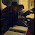## Find Minimum in Rotated Sorted Array

Suppose a sorted array is rotated at some pivot unknown to you beforehand.

(i.e., 0 1 2 4 5 6 7 might become 4 5 6 7 0 1 2).

Find the minimum element.

You may assume no duplicate exists in the array.

### Analysis:

In a sorted array, the minimum is the first element. Now the array has been rotated, so we need to search the minimum element. Binary search is usually a efficient way dealing with such problems where the "sub" array has the similar structure of the "parent" array.

In this problem, there is only 1 rotation, so that there are only limited cases when we split the array using the mid-element:
1. the right part is ordered (A[mid] < A[ed])
2. the right  part is unordered (A[mid] > A[ed])
Some might say that what about the left part of the array? Note that there is only 1 rotation, which indicates that if right part is unordered, the left part of array must be ordered.

Considering the above two cases, finding the minimum is now becoming a simple binary search with slight modifications. Details can be seen in the following code.

### Code(C++):

class Solution {
public:
void bs(vector<int> &num, int st,int ed, int &res){
if (st>ed){ return; }
else {
int mid = st+(ed-st)/2; //get middle index
if (num[mid]<num[ed]){  // right part ordered
res = min(res,num[mid]); //get right part min
bs(num,st,mid-1,res); //search left part
} else {  //right part unordered
res = min(res,num[st]); //get left part min
bs(num, mid+1, ed,res); //search right part
}
}
}
int findMin(vector<int> &num) {
int n = num.size();
int res = num;
bs(num,0,n-1,res);
return res;
}
};


### Code(Python):

class Solution:
# @param num, a list of integer
# @return an integer
def bs(self, num, st, ed, res):
if st > ed:
return res
else:
mid = st + (ed - st)/2
if num[mid] < num[ed]:
res = min(res, num[mid])
return self.bs(num, st, mid-1, res)
else:
res = min(res, num[st])
return self.bs(num, mid+1, ed, res)

def findMin(self, num):
n = len(num)
res = num
return self.bs(num, 0, n-1, res)


#### 1 comment:

1.